# 14 FM_Generation.pdf

28. May 2023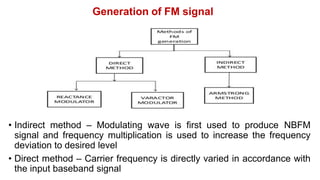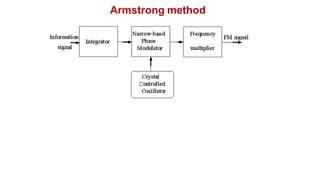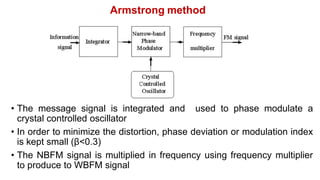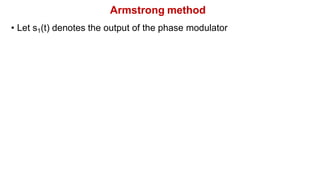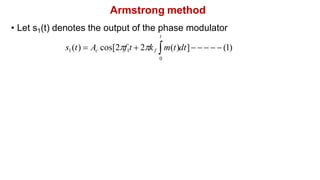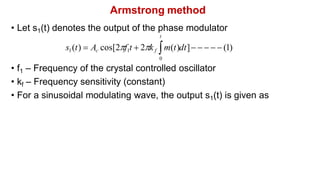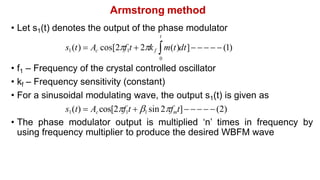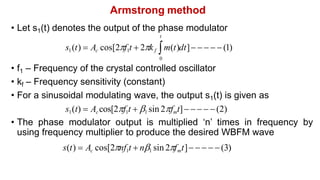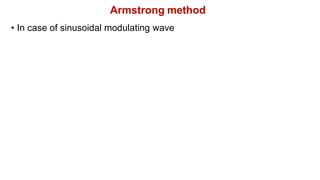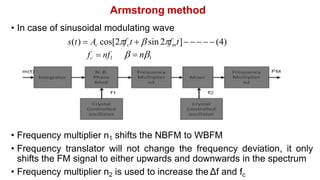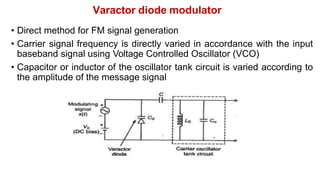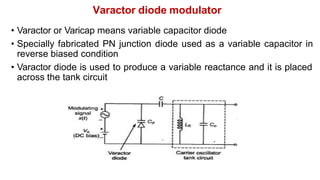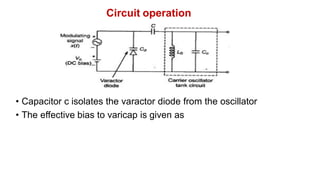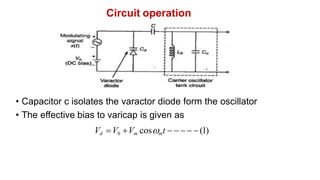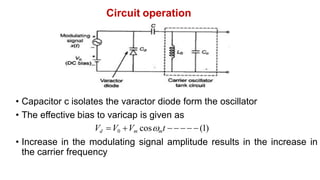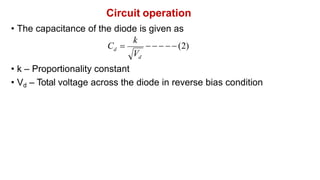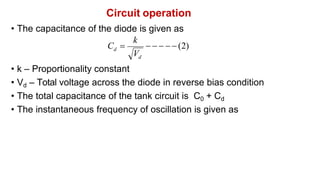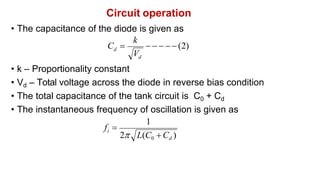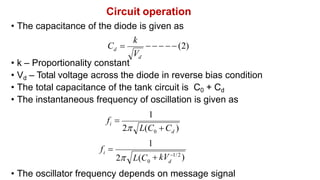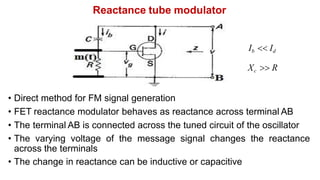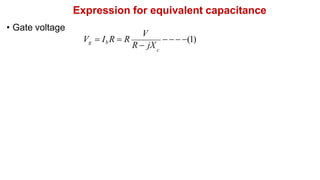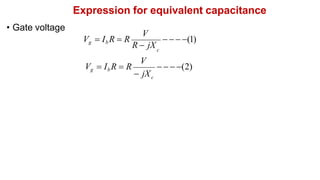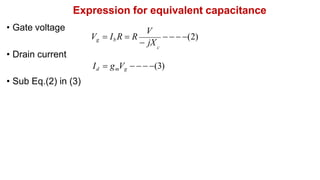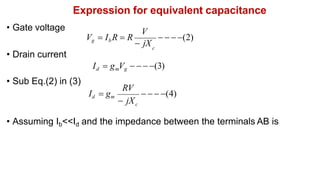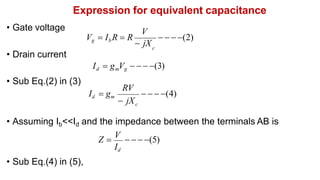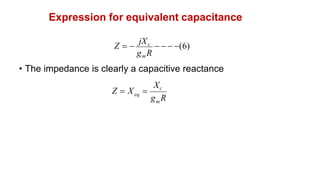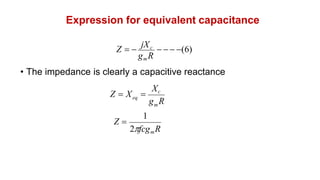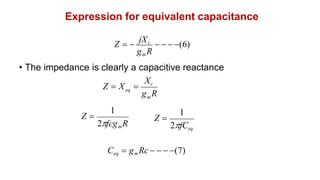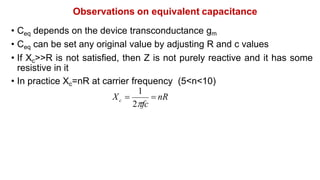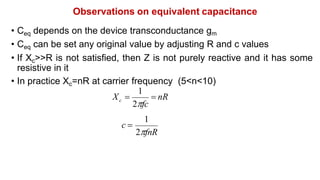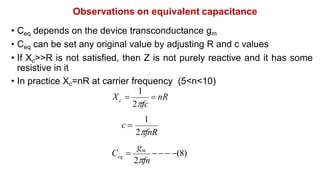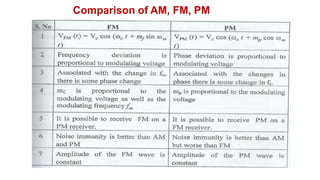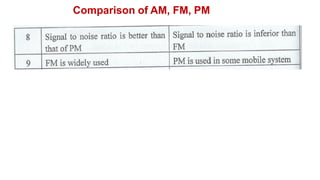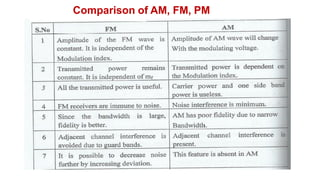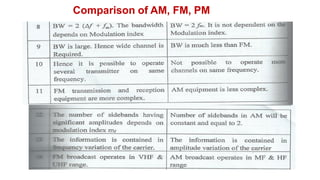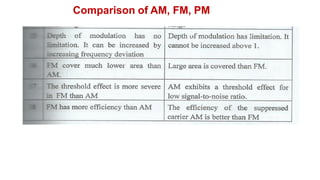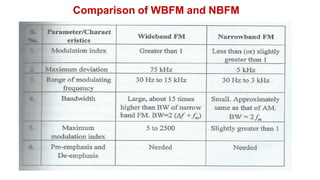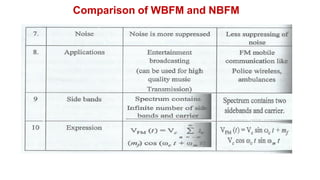1 von 38

### 14 FM_Generation.pdf

• 1. Generation of FM signal • Indirect method – Modulating wave is first used to produce NBFM signal and frequency multiplication is used to increase the frequency deviation to desired level • Direct method – Carrier frequency is directly varied in accordance with the input baseband signal
• 2. Armstrong method
• 3. Armstrong method • The message signal is integrated and used to phase modulate a crystal controlled oscillator • In order to minimize the distortion, phase deviation or modulation index is kept small (β<0.3) • The NBFM signal is multiplied in frequency using frequency multiplier to produce to WBFM signal
• 4. Armstrong method • Let s1(t) denotes the output of the phase modulator
• 5. Armstrong method • Let s1(t) denotes the output of the phase modulator t s1(t)  Ac cos[2f1t  2kf m(t)dt]     (1) 0
• 6. Armstrong method • Let s1(t) denotes the output of the phase modulator t s1(t)  Ac cos[2f1t  2kf m(t)dt]     (1) 0 • f1 – Frequency of the crystal controlled oscillator • kf – Frequency sensitivity (constant) • For a sinusoidal modulating wave, the output s1(t) is given as
• 7. Armstrong method • Let s1(t) denotes the output of the phase modulator t s1(t)  Ac cos[2f1t  2kf m(t)dt]     (1) 0 • f1 – Frequency of the crystal controlled oscillator • kf – Frequency sensitivity (constant) • For a sinusoidal modulating wave, the output s1(t) is given as s1(t)  Accos[2f1t  1 sin 2fmt]    (2) • The phase modulator output is multiplied ‘n’ times in frequency by using frequency multiplier to produce the desired WBFM wave
• 8. Armstrong method • Let s1(t) denotes the output of the phase modulator t s1(t)  Ac cos[2f1t  2kf m(t)dt]     (1) 0 • f1 – Frequency of the crystal controlled oscillator • kf – Frequency sensitivity (constant) • For a sinusoidal modulating wave, the output s1(t) is given as s1(t)  Accos[2f1t  1 sin 2fmt]    (2) • The phase modulator output is multiplied ‘n’ times in frequency by using frequency multiplier to produce the desired WBFM wave s(t)  Ac cos[2nf1t  n1 sin 2fmt]    (3)
• 9. Armstrong method • In case of sinusoidal modulating wave
• 10. Armstrong method • In case of sinusoidal modulating wave s(t)  Ac cos[2fct   sin 2fmt]    (4) • Frequency multiplier n1 shifts the NBFM to WBFM • Frequency translator will not change the frequency deviation, it only shifts the FM signal to either upwards and downwards in the spectrum • Frequency multiplier n2 is used to increase the Δf and fc   n1 fc  nf1
• 11. Varactor diode modulator • Direct method for FM signal generation • Carrier signal frequency is directly varied in accordance with the input baseband signal using Voltage Controlled Oscillator (VCO) • Capacitor or inductor of the oscillator tank circuit is varied according to the amplitude of the message signal
• 12. Varactor diode modulator • Varactor or Varicap means variable capacitor diode • Specially fabricated PN junction diode used as a variable capacitor in reverse biased condition • Varactor diode is used to produce a variable reactance and it is placed across the tank circuit
• 13. Circuit operation • Capacitor c isolates the varactor diode from the oscillator • The effective bias to varicap is given as
• 14. Circuit operation • Capacitor c isolates the varactor diode form the oscillator • The effective bias to varicap is given as Vd V0 Vm cosmt     (1)
• 15. Circuit operation • Capacitor c isolates the varactor diode form the oscillator • The effective bias to varicap is given as Vd V0 Vm cosmt     (1) • Increase in the modulating signal amplitude results in the increase in the carrier frequency
• 16. Circuit operation • The capacitance of the diode is given as • k – Proportionality constant • Vd – Total voltage across the diode in reverse bias condition     (2) d d C  V k
• 17. Circuit operation • The capacitance of the diode is given as • k – Proportionality constant • Vd – Total voltage across the diode in reverse bias condition • The total capacitance of the tank circuit is C0 + Cd • The instantaneous frequency of oscillation is given as     (2) d d C  V k
• 18. Circuit operation • The capacitance of the diode is given as • k – Proportionality constant • Vd – Total voltage across the diode in reverse bias condition • The total capacitance of the tank circuit is C0 + Cd • The instantaneous frequency of oscillation is given as     (2) d d C  V k 2 L(C0 Cd ) 1 i f 
• 19. Circuit operation • The capacitance of the diode is given as • k – Proportionality constant • Vd – Total voltage across the diode in reverse bias condition • The total capacitance of the tank circuit is C0 + Cd • The instantaneous frequency of oscillation is given as • The oscillator frequency depends on message signal     (2) d d C  V k 1 0 d i f  2 L(C C ) 1 0 d  kV 1/2 ) 2 L(C i f 
• 20. Reactance tube modulator Ib  Id Xc  R • Direct method for FM signal generation • FET reactance modulator behaves as reactance across terminal AB • The terminal AB is connected across the tuned circuit of the oscillator • The varying voltage of the message signal changes the reactance across the terminals • The change in reactance can be inductive or capacitive
• 21. Expression for equivalent capacitance • Gate voltage    (1) c V Vg  Ib R  R R  jX
• 22. Expression for equivalent capacitance • Gate voltage    (1) c V Vg  Ib R  R R  jX    (2)  jX Vg  Ib R  R c V
• 23. Expression for equivalent capacitance • Gate voltage • Drain current Id  gmVg    (3) • Sub Eq.(2) in (3) c V Vg  Ib R  R  jX    (2)
• 24. Expression for equivalent capacitance • Gate voltage • Sub Eq.(2) in (3) • Assuming Ib<<Id and the impedance between the terminals AB is c • Drain current Id  gmVg    (3) V Vg  Ib R  R  jX    (2)    (4)  jX Id  gm c RV
• 25. Expression for equivalent capacitance • Gate voltage • Sub Eq.(2) in (3) • Sub Eq.(4) in (5), c • Drain current Id  gmVg    (3) V Vg  Ib R  R  jX    (2)    (4)  jX Id  gm c RV Id • Assuming Ib<<Id and the impedance between the terminals AB is Z  V    (5)
• 26. Expression for equivalent capacitance gmR • The impedance is clearly a capacitive reactance Z   jXc    (6) g R Xc m eq Z  X 
• 27. Expression for equivalent capacitance gmR • The impedance is clearly a capacitive reactance Z   jXc    (6) 1 2fcgmR Z  g R Xc m eq Z  X 
• 28. Expression for equivalent capacitance gmR • The impedance is clearly a capacitive reactance Z   jXc    (6) 1 2fcgmR Z  1 2fCeq Z  Ceq  gmRc    (7) g R Xc m eq Z  X 
• 29. Observations on equivalent capacitance • Ceq depends on the device transconductance gm • Ceq can be set any original value by adjusting R and c values • If Xc>>R is not satisfied, then Z is not purely reactive and it has some resistive in it • In practice Xc=nR at carrier frequency (5<n<10)  nR 1 2fc c X 
• 30. Observations on equivalent capacitance • Ceq depends on the device transconductance gm • Ceq can be set any original value by adjusting R and c values • If Xc>>R is not satisfied, then Z is not purely reactive and it has some resistive in it • In practice Xc=nR at carrier frequency (5<n<10)  nR 1 2fc c X  1 2fnR c 
• 31. Observations on equivalent capacitance • Ceq depends on the device transconductance gm • Ceq can be set any original value by adjusting R and c values • If Xc>>R is not satisfied, then Z is not purely reactive and it has some resistive in it • In practice Xc=nR at carrier frequency (5<n<10)  nR 1 2fc c X  1 2fnR c     (8) gm 2fn C  eq
• 32. Comparison of AM, FM, PM
• 33. Comparison of AM, FM, PM
• 34. Comparison of AM, FM, PM
• 35. Comparison of AM, FM, PM
• 36. Comparison of AM, FM, PM
• 37. Comparison of WBFM and NBFM
• 38. Comparison of WBFM and NBFM